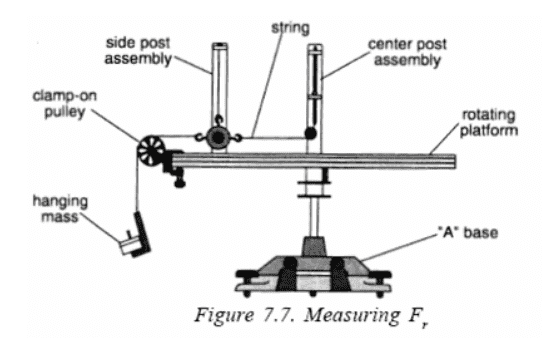# Circular Motion Pre-Lab

doggydan42

## Homework Statement

The pre-lab is based on the following lab: http://iws.collin.edu/mbrooks/physics/experiments/PHYS 2425 Exp 7 Manual.pdf

Moderator edit: Extract of an image from the PDF showing the mechanism under discussion:1. Draw two free-body diagrams for the rotating mass in teh experiment, showing all the forces acting on it when (a) it is revolving at radius r and is attached to the Center Post spring, and (b) it is revolving while hanging but not attached to the spring.

2. Why is it necessary to level the rotating platform? How would the motion of the revolving mass be affected if the base were not level? Explain.

3. Why is it important that the string attached to the revolving mass be parallel to the platform? Explain.

4. A mass m=100g is attached to a spring connected to a rotating platform. The platform is then rotated such that the centripetal force acting on the mass is supplied entirely be the spring. The period of rotation T is measured for each of a series of rotating raii r, while keeping the spring force constant. The data is displayed in the graph below ( y=.8626x, the y is T^2 in s^2 and the x is r in meters).
a) From this graph, what is the force of the spring?
b) What would be the slope of the graph if the rotating mass was reduced to 50 g?

## Homework Equations

Fc = mac
If T is the period of rotation, then v = 2πr/T

## The Attempt at a Solution

1. For the string, would there only be a centripetal force that would be the tension of the string, and for the spring would there only be the centripetal force caused by the spring?

2. I understood that the rotating platform needed to be leveled since the lab tables are not leveled. Would the motion be affected because it would be at an angle because of gravity?

4.
Solution attempt: To find the force, I thought that the problem was looking for the centripetal force. So Fc = mac = mv2/r = m(2πr/T)2/r = 4π2rm/T2
I then used the function, and set r = 1, so T2 = .8626.
I solved for the force to be 183.02 N

Solution attempt: I used the same equation, but substituted T2 for sr, where s is the slope of the function.
So,
Fc= 4π2rm/T2 = 4π2rm/(sr) = 4π2m/s ⇔ s= 4π2m/Fc
I used the same force I found in a and used a mass of .05 kg to get .011 s2/m

Thank you in advance

Last edited by a moderator:

## Answers and Replies

Homework Helper
Gold Member
2021 Award
1. For the string, would there only be a centripetal force that would be the tension of the string, and for the spring would there only be the centripetal force caused by the spring?
What do you think? We are not grading you, so draw the free body diagrams that you think are correct and we will point out any problems with them.

2. I understood that the rotating platform needed to be leveled since the lab tables are not leveled. Would the motion be affected because it would be at an angle because of gravity?
Again, what do you think? Can you picture in your mind what the motion would look like if the platform were not level?

4.
Solution attempt: To find the force, I thought that ...
You have the graph, we don't. We cannot help you figure this out if we don't know what it looks like.

Homework Helper
around 5min 40 s he starts to talk about the relationship between the centripetal force and the rotating/revolving mass.
In this case the rotating mass is the one with the three hooks above the rotating platform. This mass can swing outwards when not attached to the centre post spring (which is used to measure the centripetal force).

1. you need 2 drawings indicating the forces on the mass with the three hooks when the platform is rotating, both with the spring attached and when it is not attached

2. in this experiment we are interested in the centripetal force on the mass as a function of radius at which the mass is rotating and the mass of the rotating mass, how would gravity influence the centripetal force if the platform is rotating say in a vertical plane

3. the tension in the string from the spring need to supply the centripetal force, but if it slopes say upwards towards the central post .....

4. How are T2 and r related - write an equation in the form T2 = ..... r the ..... is then the gradient of the graph